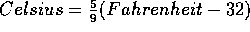Tom Kelliher, CS17

Feb. 19, 1996

# Lab Background

First, the ``nut and bolts''

A skeleton program:

```#include <iostream.h>

int main()
{
// your program is typed in here

return 0;
}
```

To declare a floating point variable:

```float variableName;
```

To input a number:

```cin >> variableName;   // variableName must have been declared earlier
```

To output a variable:

```cout << variableName;   // variableName must have been declared earlier
```

To output a variable and start a new line:

```// variableName must have been declared earlier
cout << variableName << end;
```

To output a string literal:

```cout << "Enter a number: ";
```

Some arithmetic operators:

• - --- subtraction
• * --- multiplication
• / --- division
• = --- to assign a new value to a variable
• ( ) --- to modify the order of evaluation

Conversion equation: